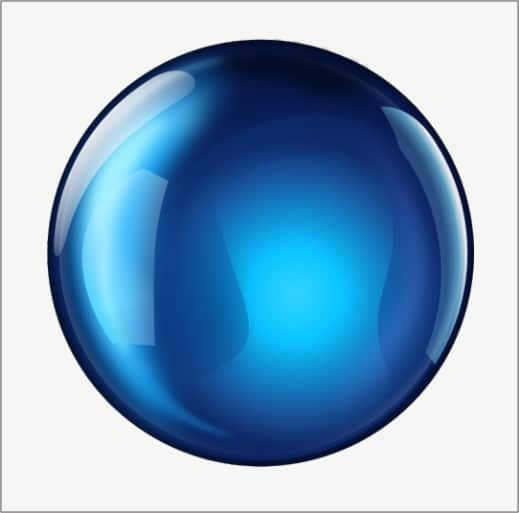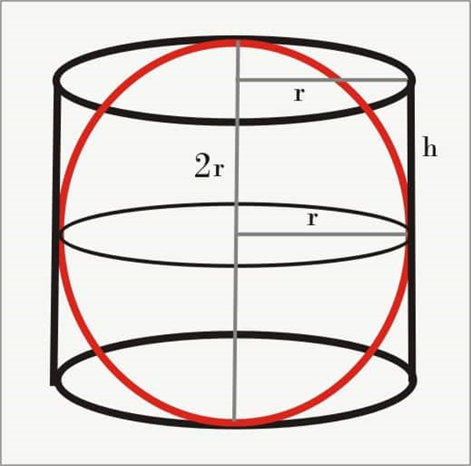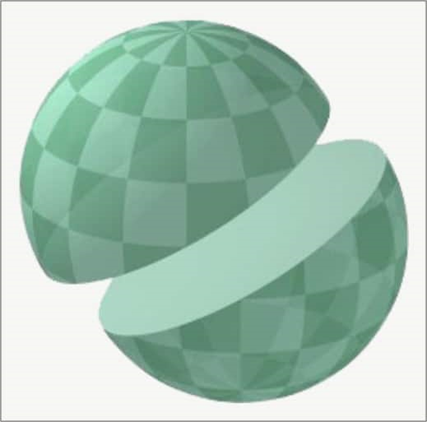Area of Hemisphere

Area of Hemisphere - Surface and Volume of Area of Hemisphere

A sphere is a three dimensional version of a circle. The definition of a sphere is the collection of all points in space that are equidistant from a given fixed point known as the centre. A ring and a ball are similar in a sense. Both are round, but can we say they are the same shape? Clearly not. One is a sphere the other is a circle.

Circles and spheres are both studied using their radii. Then what is the difference between them? A circle can be drawn on paper, but a sphere cannot.

Diagram of a SphereA circle is the set of all the points in a plane that are equidistant from a given fixed point, known as its centre. The distance between any point on the circle and its centre is known as its radius. Any line segment passing through the centre of the circle and having its two endpoints on the circle is known as its diameter. If we spin the circle keeping its diameter fixed, the object formed in 3D space is a sphere.

Surface Area

For finding the surface area of any round thing we can do this: A ball of any game is the best case of a sphere. Take a ball and insert a nail into it. Tie a string from the nail and wind it over the ball. Wind the string in such a way, that the two layers of string don't cover one another. We have to cover the ball with only one layer of string. On achieving this, use pins to keep the strings flawless. Spread the entire ball along these lines. Then mark the beginning and end points of the string. Estimate the length of the string used. The width of the ball gives us the diameter of the ball. With the same diameter, draw four circles on plain paper. With the assistance of the string that was utilized to cover the ball, fill the circles on paper successively. You will see that the string used to cover the ball, covers the four circles on paper. This conveys to us that the surface area of the ball is equal to the sum of the areas of the four circles. Consequently, the surface area of a sphere = 4 × area of a circle = 4 × πr2. So,

SA = 4πr2

Examples

Example 1: What is the total surface area of a sphere whose radius is 5.5 meters?
Solution:
Given that,
r = 5.5 m

Surface area of the sphere
SA = 4 × π × r2
SA = 4 × π × (5.5)2
SA = 4 × 3.14 × 30.25
SA = 379.94 m2

The surface area of the sphere is 379.94 m2.

Example 2: A ball has a surface area of 2464 cm2. Find the radius of the ball [π = 22/7].

Solution:
SA = 4 × π × r2
r2 = SA/(4π)
r2 =2464/(4π)
r2 =196
r = √(196)
r = 14 cm

Example 3: Find the surface area of a sphere whose radius is 18 cm. [π = 3.14]

Solution:
r = 18 cm
SA = 4πr2
SA = 4π182
SA = 4π × 324
SA = 4069.44 cm2

The surface area of the sphere is 4069.44 cm2.

Example 4: Find the surface area of a sphere whose radius is given as r = 11 cm.

Solution:
SA = 4πr2
SA = 4 × 3.14 × 112
SA = 1519.76

The surface area of sphere is 1519.76 cm2.

Example 5: A hemisphere has a radius of 8.3 cm. Find its curved surface area.

Solution:
r = 8.3 cm

The surface area of half of the sphere without the base is calculated as follows.
SA = 2πr2
SA = 2π × 8.32
SA = 432.62

The surface area of the half of the sphere is 432.62 cm2.

Example 6: Find the surface area of a sphere whose radius is 6 cm.

Solution:
SA = 4πr2
SA = 4π × 62
SA = 4π × 36
SA = 452 cm2

Volume

For finding the volume of a circular body we utilize the Archimedes rule. What is Archimedes guideline? As indicated by the Archimedes rule, when a solid figure is drenched in a compartment loaded up with water, at that point the volume of water that floods from the holder is equivalent to the volume of that solid figure. Archimedes rule essentially gives you the volume, however following this guideline is painstaking. For finding the equation for the volume of the sphere, we embed the round body in a barrel-shaped holder.The figure above demonstrates a circular body inside a cylindrical compartment. We see that the radius of the round bases of the cylinder is equal to the radius of the spherical body. What's more, since the spherical body contacts the top and base of the compartment, its diameter is equal to the height of the holder.

The volume of a circular body is thought to be 2/3 of the round and hollow cylinder, which gives us the following conditions.
V of sphere = 2/3 V of chamber or 2/3 πr2h.
But since h = 2r, we have the following.
V of sphere = 2/3 πr2(2r) = 4/3 πr3

Volume of a sphere = 4/3 πr3

Hemisphere

A hemisphere is the half piece of a sphere. If we cut the sphere into two equal parts, each part will have a curved surface as well as a flat surface. So, there are two types of areas associated with a hemisphere, which are the curved surface area and the total surface area, which is the sum of the curved and flat surface areas. Hemispheres can either be of a solid kind or can be hollow.Diagram of a hemisphere

Elements of a hemisphere

• 1. The radius is a line that connects the centre of a sphere with a point on the circumference.

• 2. The diameter is double the line segment from one side of the boundary to the other through the centre.

• 3. π is the symbol we use for pi, which is the ratio of the circumference of a circle to its diameter. π is approximately 3.14.

• The surface area of a hemisphere

The surface area of a sphere is determined by the formula 4πr2.

Now, since a hemisphere is half of a sphere, the surface area of the hemisphere ought to be 2πr2. However, as we are aware that a hemisphere additionally has a flat surface, we divide the total surface area of the hemisphere into two parts, which are the curved and flat surface areas.

The curved surface area of a hemisphere is half of the surface area of a sphere.
= ½ of (surface area of the circle)
= ½ of 4πr2
= 2πr2

Now, to find the total surface area of a hemisphere we additionally need to determine the surface area of the circular surface
of the flat base of the given hemisphere, which is equal to πr2.

Subsequently, total surface area of a hemisphere = flat surface area + curved surface area

Hence, it will be as follows.
total surface area = πr2 + 2πr2 = 3πr2

Practical examples of calculating the area of a hemisphere

Ex: Find the surface area of a hemisphere with radius 10 cm.

Solution:

Since it isn't mentioned whether we have to find the total surface area of curved surface rea, let us compute both

As we know,
curved surface area of hemisphere = 2πr2 = 2 x 3.14 x 10 x 10 = 628 cm2
Subsequently, total surface area of a hemisphere = 3πr2 = 3 x 3.14 x 10 x 10 = 942 cm2

Ex: Find the radius of a hemisphere whose the total surface area is 1000cm2?

Solution:
total surface area of a hemisphere = 3πr2
1000 = 3 x 3.14 x r2
r2  = 1000/9.42
r2  =  106.16
r  = 10.30 cm

Ex: If the radius of a hemisphere is tripled, what will be its impact on its total surface area?

Assume that the radius is 1 unit
Now, total surface area = 3πr2 = 3π

In the case where the radius is tripled, the total surface area will be = 3 x π x 32 = 27π

In this way, the surface area of half of the sphere will increase by a factor of 9.

Ex: If the radius of a hemispherical balloon increases from 5 cm to 10 cm as air is being pumped into it, find the ratio of the surface areas of the new balloon to its original.

Solution:
Beginning hemisphere,
r = 5cm
total surface area = 3πr2 = 3 x π x 52 =  75π cm2
In the case where the radius is 10 cm,
total surface area = 3πr2 = 3 x π x 102 = 300 π cm2
Therefore the ratio is as follows.
⇒ S1 : S2 = 75π : 300 π = 1 : 4